# ML | One Hot Encoding of datasets in Python

Sometimes in datasets, we encounter columns that contain numbers of no specific order of preference. The data in the column usually denotes a category or value of the category and also when the data in the column is label encoded. This confuses the machine learning model, to avoid this the data in the column should be One Hot encoded.

## One Hot Encoding –

It refers to splitting the column which contains numerical categorical data to many columns depending on the number of categories present in that column. Each column contains “0” or “1” corresponding to which column it has been placed.

For example :

Consider the data where fruits and their corresponding categorical value and prices are given.

Fruit Categorical value of fruit Price
apple 1 5
mango 2 10
apple 1 15
orange 3 20

The output after one hot encoding the data is given as follows,

apple mango orange price
1 0 0 5
0 1 0 10
1 0 0 15
0 0 1 20

Below is the Implementation in Python –

Example 1:

The following example is the data of zones and credit scores of customers, the zone is a categorical value which needs to be one hot encoded.

 `# Program for demonstration of one hot encoding ` ` `  `# import libraries ` `import` `numpy as np ` `import` `pandas as pd ` ` `  `# import the data required ` `data ``=` `pd.read_csv(r``"../../onehotenc_data.csv"``) ` `print``(data) `

Output: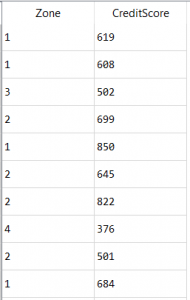To one hot encode the zone column –

 `# importing one hot encoder from sklearn ` `# There are changes in OneHotEncoder class ` `from` `sklearn.preprocessing ``import` `OneHotEncoder ` `from` `sklearn.compose ``import` `ColumnTransformer ` `  `  `# creating one hot encoder object with categorical feature 0 ` `# indicating the first column ` `columnTransformer ``=` `ColumnTransformer([(``'encoder'``, ` `                                        ``OneHotEncoder(), ` `                                        ``[``0``])], ` `                                      ``remainder``=``'passthrough'``) ` ` `  `data ``=` `np.array(columnTransformer.fit_transform(data), dtype ``=` `np.``str``) `

Output: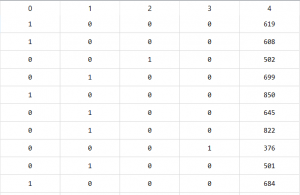The output contains 5 columns, one column for the price, and the remaining 4 columns representing the 4 zones.

Example 2:

One hot encoder only takes numerical categorical values, hence any value of string type should be label encoded before one-hot encoded.
The below example has the data of geography and gender of the customers which has to be label encoded first.

 `# importing libraries ` `import` `numpy as np ` `import` `pandas as pds ` ` `  `# After importing the required data ` `print``(data) `

Output: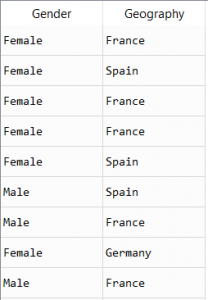Label encoding the data –

 `# label encoding the data ` `from` `sklearn.preprocessing ``import` `LabelEncoder ` ` `  `le ``=` `LabelEncoder() ` ` `  `data[``'Gender'``]``=` `le.fit_transform(data[``'Gender'``]) ` `data[``'Geography'``]``=` `le.fit_transform(data[``'Geography'``]) `

Output: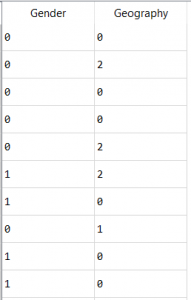One Hot Encoding Gender and Geography Columns –

 `# importing one hot encoder from sklearn ` `from` `sklearn.preprocessing ``import` `OneHotEncoder ` ` `  `# creating one hot encoder object by default ` `# entire data passed is one hot encoded ` `onehotencoder ``=` `OneHotEncoder() ` ` `  `data ``=` `np.array(columnTransformer.fit_transform(data), dtype ``=` `np.``str``) `

Output: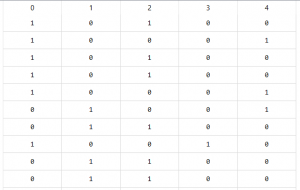The output contains 5 columns, 2 columns representing the gender, male and female, and the remaining 3 columns representing the countries France, Germany, and Spain.

Note :

1. The one hot encoder does not accept 1-dimensional array or a pandas series, the input should always be 2 Dimensional.
2. The data passed to the encoder should not contain strings.

Attention geek! Strengthen your foundations with the Python Programming Foundation Course and learn the basics.

To begin with, your interview preparations Enhance your Data Structures concepts with the Python DS Course.

My Personal Notes arrow_drop_upCheck out this Author's contributed articles.

If you like GeeksforGeeks and would like to contribute, you can also write an article using contribute.geeksforgeeks.org or mail your article to contribute@geeksforgeeks.org. See your article appearing on the GeeksforGeeks main page and help other Geeks.

Please Improve this article if you find anything incorrect by clicking on the "Improve Article" button below.

Improved By : architjainbansal

Article Tags :
Practice Tags :

8

Please write to us at contribute@geeksforgeeks.org to report any issue with the above content.